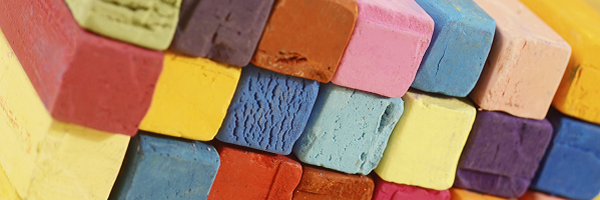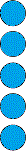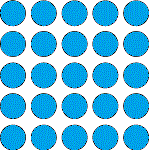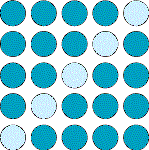# Odd SquareThink of a number.
Square it.
Subtract your starting number.

Is the number you're left with odd or even?

Try with other numbers.

What do you notice?

Can you use the diagrams to explain what you have noticed?Think of a number.
Cube it.
Subtract your starting number.

Try it a few times and record your results.

What do you notice this time?

Is there a diagram or model that can help you explain what you have noticed?